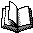Source code

• Imlib A small collection of C++ image processing programs, with Makefiles for Linux, but the code should work for both windows and Linux (may take some tweaking, but should run on visual studio express 2013). Please note that the code is nowhere near a toolbox or a framework, it's mostly for sharing various algorithms. If you want a proper computer vision toolbox, openCV is the natural choice.

• A spectral fitting package (source code in C++) for Linux/Cygwin. Uses unix sed/awk and Gnuplot as visual feedback.

• "Mathutil" contains various mathematical functions in C++ that I have written myself and copied from others. Examples include cylindrical and spherical Bessel functions (J,Y,K,I), a random number generator with period 2**144 and an approximation of Gaussian probability phi(x) and many other functions. Credits to authors and URL:s are given in the code. In many cases the URL:s in the code don't work but I neverteheless hope it will clear who the real author is.

mathutil.h
mathutil.cpp

The program "rempol" fits an n:th order polynomial and removes it from the data. The method used is a discrete orthogonal polynomial Fourier (OPF) expansion (which diagonalizes the normal equations) for numerical stability. The program is useful for example when removing wiggly baselines in signals. The OPF expansion gives much more stable results, and large polynomial orders may be used without loosing numerical stability (be careful about overfitting).

polyfit.h
polyfit.cpp
rempol.cpp

Sometimes data smoothing is necessary while retaining the global max-and-min structure of data, such as in the treatment of spectra. The Savitzky-Golay filter satisfies these critera and is based on polynomial interpolation expressed as a FIR-filter. The programs use the newmat11library.

savgolayfilter.rar

Here is sample openCV code that given a calibrated camera (specified in an XML-file) will give the current estimated pose from a chessboard. You can save the 3x4 projection matrix by pressing escape. The input is an xml file with board specifications, and a calibration file (K-matrix); the chessboard file is given below below. Please note that you need a separate program to extract the camera-matrix and radial distortion coeffs, see the openCV documentation. The code has been tested on raspberry Pi 2 (Linux) and standard windows 7/10 laptop.chesspose2.cpp
board.xml
calib.xml
chessboard